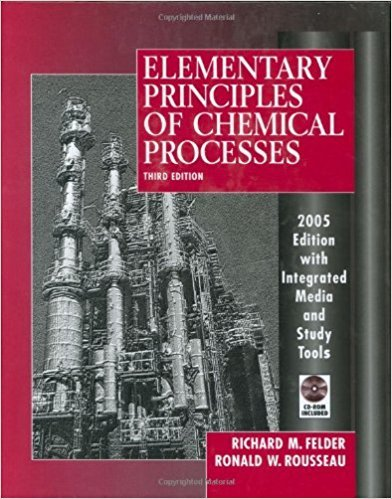×
Get Full Access to Elementary Principles Of Chemical Processes - 3 Edition - Chapter 8 - Problem 8.64
Get Full Access to Elementary Principles Of Chemical Processes - 3 Edition - Chapter 8 - Problem 8.64

×

# A mixture containing 35.0 mole% n-butane and the balanceISBN: 9780471687573 143

## Solution for problem 8.64 Chapter 8

Elementary Principles of Chemical Processes | 3rd Edition

• Textbook Solutions
• 2901 Step-by-step solutions solved by professors and subject experts
• Get 24/7 help from StudySoup virtual teaching assistantsElementary Principles of Chemical Processes | 3rd Edition

4 5 1 395 Reviews
18
1
Problem 8.64

A mixture containing 35.0 mole% n-butane and the balance isobutane at lOoC enters a he3t exchanger at a rate of 24.5 kmollh and a pressure high enough for the mixture to be a liquid. Toe exchanger has been designed to heat and vaporize the liquid and heat the vapor mixture to lS(YC The heating fluid is a high molecular weight liquid with a constant heat capacity Cp = 2..62 kJ (kg'=C), It enters the exchanger at 21SOC and flows countercurrently to the hydrocarbon mi.xture. (a) Estimate the minimum pressure (bar) required for the hydrocarbon feed to be a liquid. ese Raoult's law and the Cox chart (Figure 6.1-4) in your calculation. (b) Assuming that the heat capacities and heats of vaporization of n-butane and isobutane are independent of pressure (so that the values in Tables B.1 and B.2 may be used), calculate the enC:ialpy change t.il (kJ/h) undergone by the hydrocarbon mixture in the heat exchanger. Show the process paths you use for n-butane and i-butane in your calculation. (Hint: Since you dont haye heat capacities for liquid n-butane and i-butane in this text, use process paths that don't rc,quire them.) (c) According to the heat exchanger design calculations. the heating fluid exit tem~rature should be 45C. Assuming that all the heat lost by the heating fluid is transferred to the hydrocarbon mixture, what is the required mass flow rate of the heating fluid. lhhf(kgib)? (d) When the heat exchanger is run with mhf equal to the value calculated in part (b)' the exit temperature of the hydrocarbon mixture is measured and found to be only 155C instead of the design value of ISOC. The process operator observes that the outside of the exchanger is hot to the touch, indicating that some of the heat lost by the heating fluid is escaping into the plant 426 Chapter 8 Balances on Nonreactive Processes instead of being transferred to the hydrocarbon mixture. After discussing the situation with a production engineer, the operator gradually increases the flow rate of the heating fluid while continuing to monitor the outlet temperature of the hydrocarbon. When the flow rate reaches 2540 kg/h, the outlet fluid temperatures level out at their design values (180C for the hydrocarbon and 45C for the heating fluid). At what rate (kJ/h) is heat being transferred from the exchanger to the plant air? (e) When the heating fluid leaves the exchanger, it passes through a heater, which raises its temperature back to 215C, and is recycled back to the exchanger. How is the profitability of the process being decreased by the heat loss from the exchanger to the surroundings? (Try to think of two costs that result from the heat loss.) (I) The engineer proposes adding more insulation to the heat exchanger, which would cut down On the heat loss and reduce the required heating fluid flow rate. What are advantages and disadvantages of the two responses to the heat loss problem (adding insulation versus increasing the heating fluid flow rate)? Which would you guess would be the preferable response in the long run, and why?

Step-by-Step Solution:
Step 1 of 3

CH 111 Notes: Week 8  If 25.0 g Cu at 90.0ᵒC is placed in 100.0 g of water at 20.0ᵒC, what will the final temperature of the water be (c p(Cu)= 24.4 J/mol*ᵒC) o Assume T final the same for both Cu and H O 2 o Assume container absorbs negligible heat → q Cu= -q H2O o 25.0 g Cu (1 mol Cu/63.55g) = 0.3934 mol Cu 100.0 g H 2 (1 mol H O/28.02 g) = 5.5494 mol H O 2 o cp(Cu)*∆T = -( c p(H2O)n*∆T) (0.3934 mol)(24.4 J/mol*ᵒC)(T – f0.0ᵒC) = -(5.5494 mol)(75.3 J/mol*ᵒC) (Tf– 20.0ᵒC) = 9.599 J/ᵒC(Tf– 90.0ᵒC) = -417.87 J/ᵒC(T –f20.0ᵒC) = 9.599 J/ᵒC(Tf) – 863.9 J = -417.87 J/ᵒC(T f – 8357 J = 427.5 J/ᵒC(Tf) = 9221 J Tf= 21.6ᵒC  A 2.50 g sample of Zn at 22.

Step 2 of 3

Step 3 of 3

##### ISBN: 9780471687573

Unlock Textbook Solution